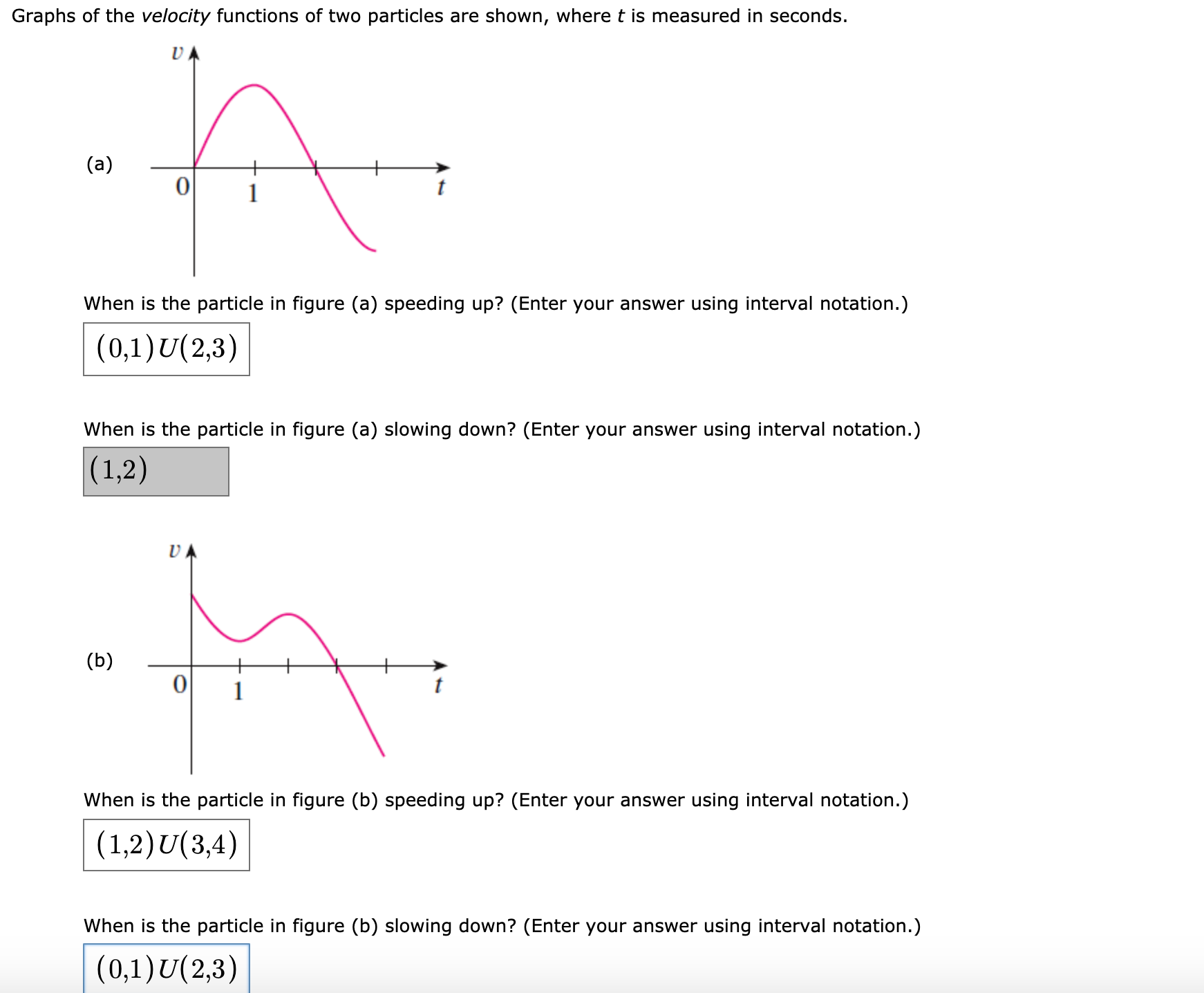# Graphs of the velocity functions of two particles are shown, where t is measured in seconds. (a) When is the particle in figure (a) speeding up? (Enter your answer using interval notation.) (0,1)U(2,3) When is the particle in figure (a) slowing down? (Enter your answer using interval notation.) (1,2) UA (b) When is the particle in figure (b) speeding up? (Enter your answer using interval notation.) (1,2)U(3,4) When is the particle in figure (b) slowing down? (Enter your answer using interval notation.) (0,1)U(2,3)

Question

I'm stuck on this question and I don't know how I should be approaching this. What should I do?help_outlineImage TranscriptioncloseGraphs of the velocity functions of two particles are shown, where t is measured in seconds. (a) When is the particle in figure (a) speeding up? (Enter your answer using interval notation.) (0,1)U(2,3) When is the particle in figure (a) slowing down? (Enter your answer using interval notation.) (1,2) UA (b) When is the particle in figure (b) speeding up? (Enter your answer using interval notation.) (1,2)U(3,4) When is the particle in figure (b) slowing down? (Enter your answer using interval notation.) (0,1)U(2,3) fullscreen

1 Rating

### Want to see this answer and more?

Experts are waiting 24/7 to provide step-by-step solutions in as fast as 30 minutes!*

*Response times may vary by subject and question complexity. Median response time is 34 minutes for paid subscribers and may be longer for promotional offers.
Tagged in
Math
Calculus

### Other